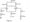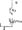# Linear Integrated Circuits and Applications February 2006

Note: Answer any FIVE full questions.

1.  (a) Give the block diagram approach for the internal structure of an opAmp and explain each block in detail.

(b) Determine voltage gain, input resistance, output resistance, maximum output voltage without clipping for the dual input balanced output differential amplifier : : as shown in Fig.l.Also determine output voltage if v^nl = 50mV(p~p) and v^n2 = 20mV(p p) at ) kHz. Draw the input and output waveforms.

2. (a) What are the ideal electrical characteristics of an opAmp?

(b) Design a zener constant current source as shown in fig.2 according to the following specifications.

i)  Emitter current Jg3 = 5mA,                                ‘

ii) IN385 zenerdiode with V% = 4.7 V and = 53mA

iii)  CA3086 transistor with /?ac = = 100 and = 0.715V

iv)  supply voltages Vs = ±9V.(c)  Define the following terms and write their typical values for //A741 op Amp,

i) Input bias current

ii)   input offset voltage

i)     Slew rate

iv)   CMRR.

3. (a) Explain the working of opAmp as a non-inverting amplifier with feedback. Also, derive an expression for’ voltage gain and input resistance.

(b) Design an opAmp Schmitt trigger circuit with UTP — 2 V, LTP — IV, supply voltage = ±15V, Vsai — ±13 V. Draw the circuit with designed values.. Draw the output waveform when the input is Ssinwt.                                                                                                             )

4   (a) Design a second order butterworth low pass filter with cut-off frequency of 2 kHz. Write the design steps.

(b) What are the advantages of active filters over passive filters?.

(c) Explain the astable multivibrator using opAmp and obtain the expression for 2\& T2 and frequency of oscillation.

5 (a) Explain with a neat functional diagram the working of 555 timer as a monostable multivibrator. Derive an expression for pulse width, Draw the waveforms.

(b) Explain the performance specifications of DAC.

6  (a) Explain the working of Binary weighted network 4-bit DAC. Derive an expression for the output voltage.

(b) Explain the working successive approximation type ADC with a neat diagram.

7.  (a) Draw a neat block diagram of PLL and explain its working.

(b) Explain how PLL can be used as on FM detector.

(c) Design a suitable circuit to provide a load current of 150 mA to a load of 8H, 10TV using 7805 regulator 1C. Determine the range of input voltage for satisfactory, operation.

8.        Write short notes on :

(a)       Sample and hold circuits

(b)       Thermal drift

(c)       V to 1 converter

(d)       Switching regulator.